# ComposeCharter

A library that contains several customizable Charts that can be used in Jecpack Compose’s `@Composables`.

## Basic Usage

```allprojects {
repositories {
...
maven { url 'https://jitpack.io' }
}
}```

```dependencies {
implementation 'com.github.nikachapo:ComposeCharter:1.0.1'
}```

### 3. Use Chart Composables:

There is one data class that can be used for drawing Charts:

`data class ChartModel(val value: Float, val color: Color, val text: String? = null)`

Note: for Animations, library does not have inner implementations, you can use common Animation approach as you would do for composables, for example:

```    var cyanPercentage by remember { mutableStateOf<Float?>(0f) }
var greenPercentage by remember { mutableStateOf<Float?>(0f) }
var yellowPercentage by remember { mutableStateOf<Float?>(0f) }

val cyanAnimatedPercentageState = animateFloatAsState(
targetValue = cyanPercentage ?: 0f,
animationSpec = tween(1000)
)

val greenAnimatedPercentageState = animateFloatAsState(
targetValue = greenPercentage ?: 0f,
animationSpec = tween(1000)
)

val yellowAnimatedPercentageState = animateFloatAsState(
targetValue = yellowPercentage ?: 0f,
animationSpec = tween(1000)
)
```

#### PieChart

```PieChart(
chartModels = listOf(
ChartModel(cyanAnimatedPercentageState.value, Color.Cyan),
ChartModel(greenAnimatedPercentageState.value, Color.Green),
ChartModel(yellowAnimatedPercentageState.value, Color.Yellow)),
chartSize = 150.dp,
elevation = 30.dp,
showCenterDot = true
)```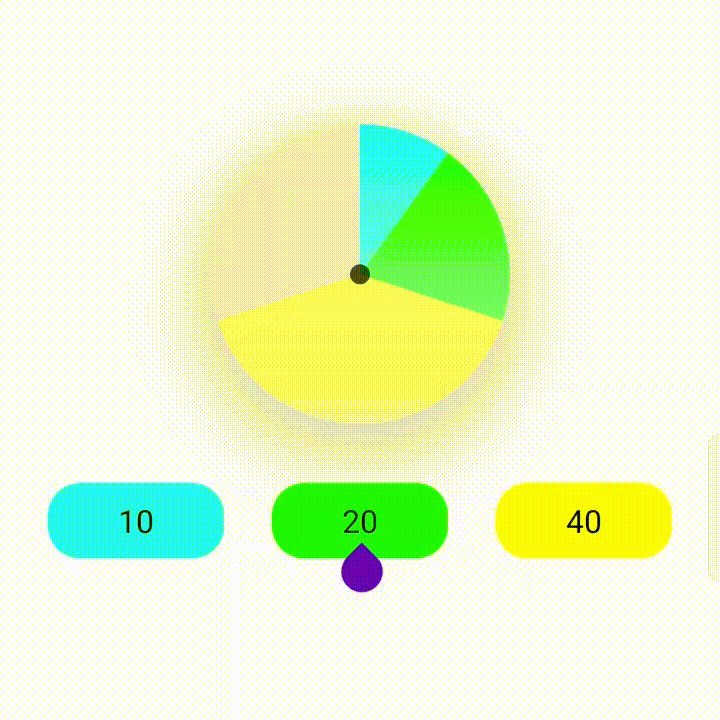#### DonutChart

```DonutChart(
chartModels = chartModels,
chartSize = 150.dp,
strokeWidth = 100f,
elevation = 30.dp,
cap = StrokeCap.Round
)```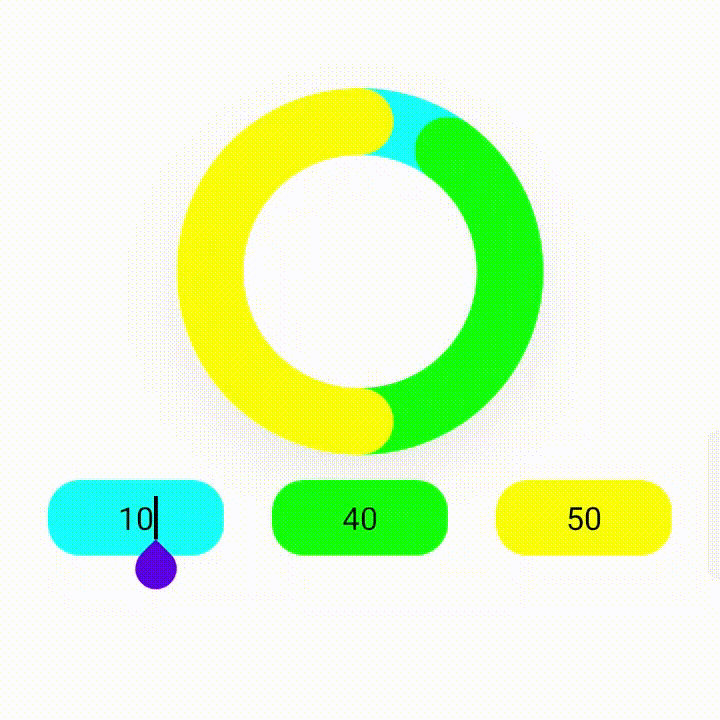#### ColumnChart

```ColumnChart(
chartModels = chartModels,
modifier = Modifier.fillMaxWidth(),
height = 150.dp,
columnWidth = 150f,
spaceWidth = 40f,
showValues = true
)```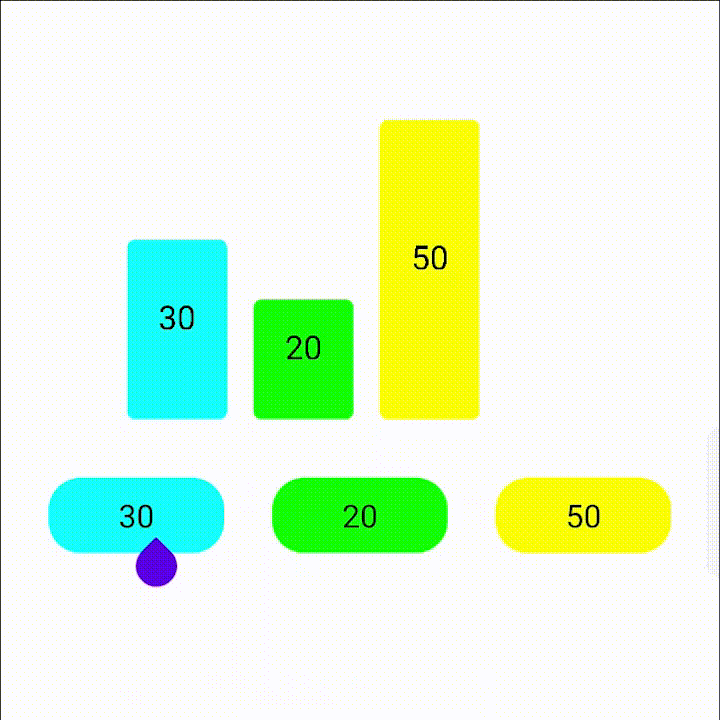#### BarChart

```BarChart(
chartModels = chartModels,
modifier = Modifier
.fillMaxWidth()
height = 200.dp
)```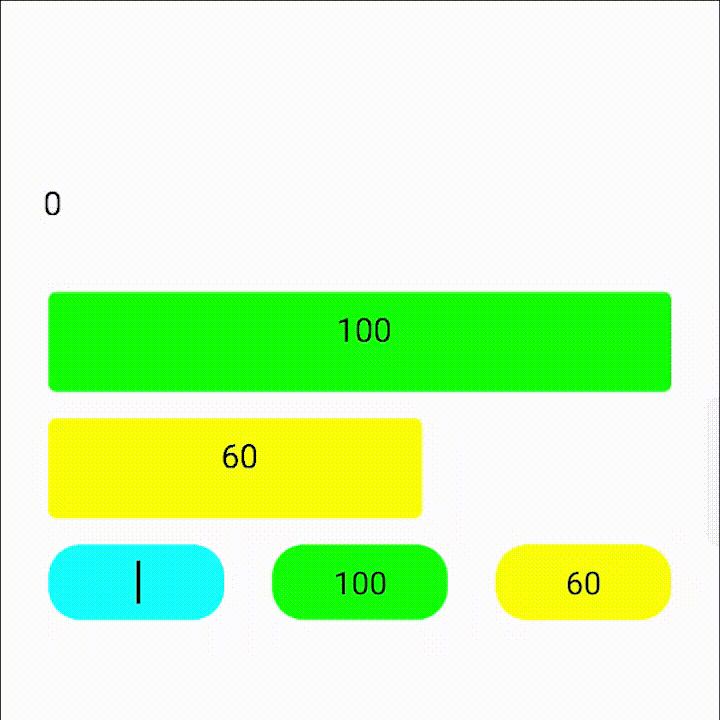#### LineChart

```LineChart(
yAxisValues = listOf(10f, 20f, 15f),
modifier = Modifier
.fillMaxWidth()
.height(200.dp)
lineWidth = 2f,
shouldAnimate = true,
shouldDrawDots = true,
lineColors = listOf(
MaterialTheme.colors.primary,
Color.Green
)
)```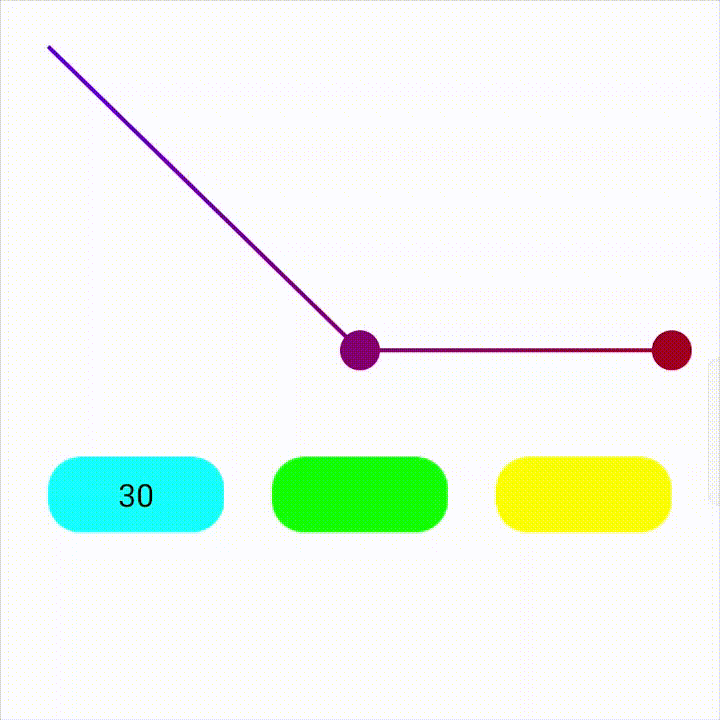#### AreaChart

```AreaChart(
yAxisValues = listOf(10f, 20f, 15f),
modifier = Modifier
.fillMaxWidth()
.height(200.dp),
spacing = 50f,
lineWidth = 2f,
fillColor = Color.Green,
graphColor = MaterialTheme.colors.primary
)```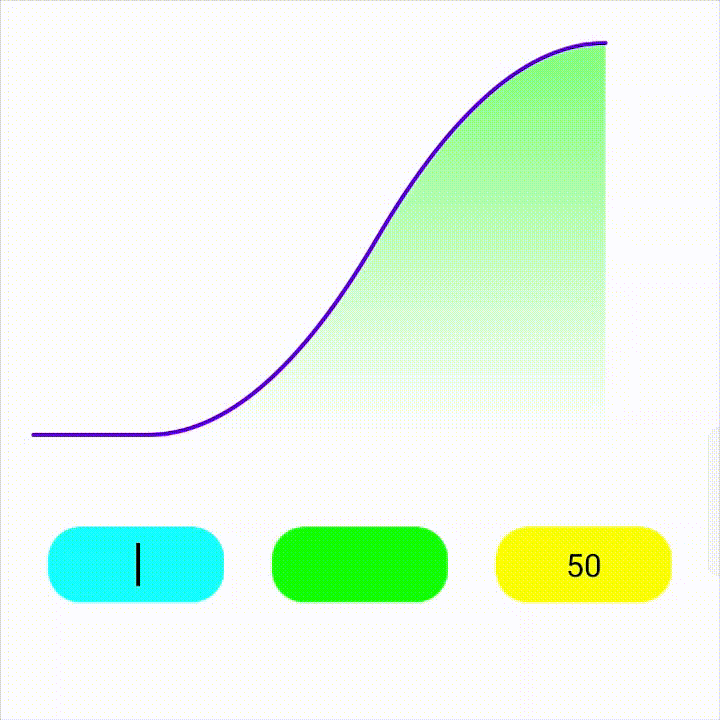View Github